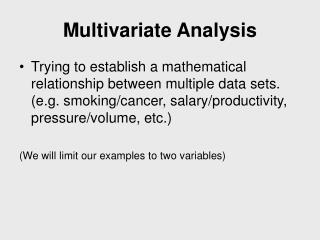# Multivariate Analysis - PowerPoint PPT PresentationDownload PresentationMultivariate Analysis

Multivariate AnalysisDownload Presentation## Multivariate Analysis

- - - - - - - - - - - - - - - - - - - - - - - - - - - E N D - - - - - - - - - - - - - - - - - - - - - - - - - - -
##### Presentation Transcript

1. Trying to establish a mathematical relationship between multiple data sets. (e.g. smoking/cancer, salary/productivity, pressure/volume, etc.) (We will limit our examples to two variables) Multivariate Analysis

2. Multivariate Analysis • Correlation: Mathematical tool used to establish a dependency between two data sets. Covariance (shown below) Product of Standard deviations Deviations from average value X and Y represent the two sets of data

3. R: Value ranges between -1 and +1. Applies only to linear systems Interpretation is subjective but in general the closer to -1 or +1 the more highly correlated is the data. Multivariate Analysis

4. Correlation Continued • Magnitude of association Correlation coefficient with a value between -1 and 1. Weak 0 - 0.3 Moderate 0.3 - 0.6 Strong 0.6 - 1.0 Discipline dependent

5. Correlation Continued • Direction Sign of the correlation coefficient Positive correlation (+) - an increase in one variable is accompanied by an increase in the other Negative correlation (-) - an increase in one variable is accompanied by an decrease in the other

6. Multivariate Analysis X Y • R close to zero

7. Multivariate Analysis • R close to +0.5

8. Multivariate Analysis • R close to +1

9. Excel Functions COVAR(array1, array2) CORREL(array1, array2) Multivariate Analysis

10. Correlation Continued • Correlation or association between two variable does not give causation • More experimentation must be done to determine causation.

11. Causation • Consistency - measurements of the same two properties yield nearly the same results • Responsiveness - Change in one variable elicits corresponding change in the other variable • Mechanism - finding a valid and reasonable explanation for the cause and effect between the pair of variables

12. Establishing Causation Continued • A valid and reasonable explanation establishes the sequential ordering of events and, thus, provides the rationale for the existence of such a relationship. • Cause must precede effect, otherwise there is no causality